#### How to Calculate Straight Line Depreciation

Straight line depreciation is a method of calculating the decline in an asset’s value over time in equal amounts. In this Excel lesson, you will learn how to calculate Stright Line Depreciation.

## Straight Line Depreciation in Excel

The asset:

• it is 1 million of dollars worth
• salvage value is \$750 000
• life of the asset is 10 years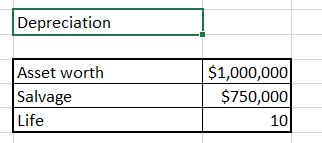### SLN formula

To calculate straight line depreciation, we will use the SLN Excel formula.

Syntax of Straight Line Depreciation formula is:

• cost
• salvage
• life

Luckily, we already have all data we need.

## Straight line depreciation calculator

To calculate yearly depreciation use =SLN(\$C\$4,\$C\$5,\$C\$6) formula.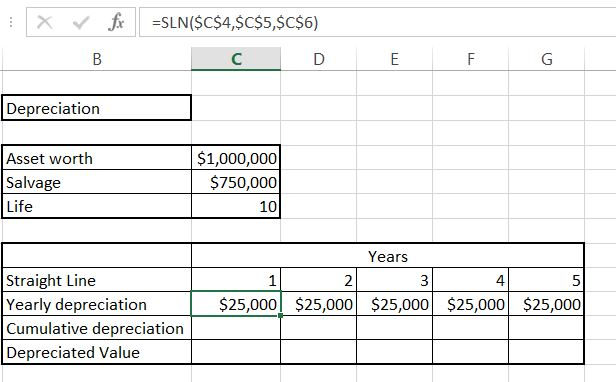Absolute reference is needed because the data will not change in the table. For every year it is the same amount of \$25 000 of yearly cost.

Cumulative depreciation is just =C11+D10. Drag it right to calculate all of them. This is just current year value plus sum of previous ones.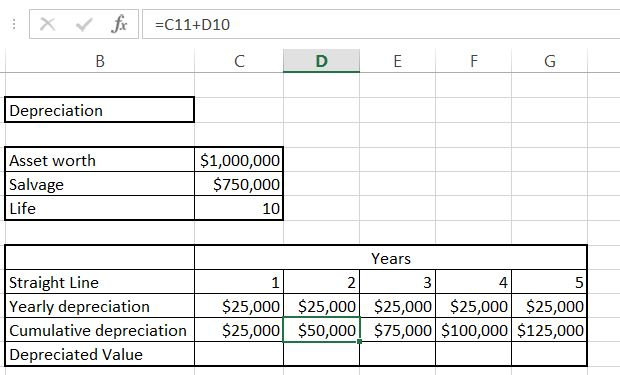Deprecated value is =\$C\$4-C11 which is the starting value reduced by cumulative depreciation.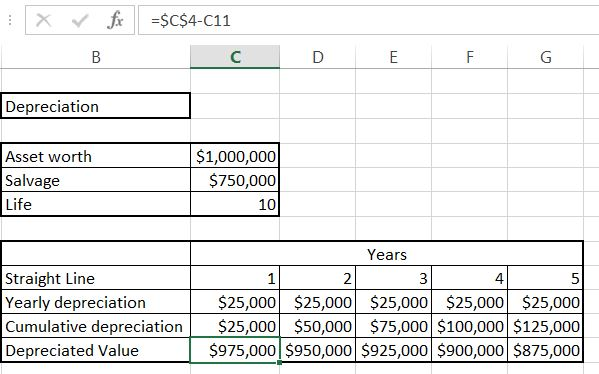This is the Straight Line Depreciation formula and the easiest way to calculate Straight Line Depreciation in Excel.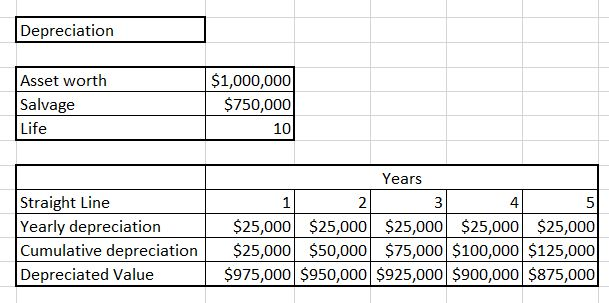The difference between straight line depreciation and declining balance is that for straight line depreciation, yearly depreciation is the same value, while for declining balance, it is different (based on percentage).

Note: The above formula assumes that the straight line depreciation is calculated on a yearly basis. If you want to calculate the depreciation for a different time period, you will need to adjust the formula accordingly.